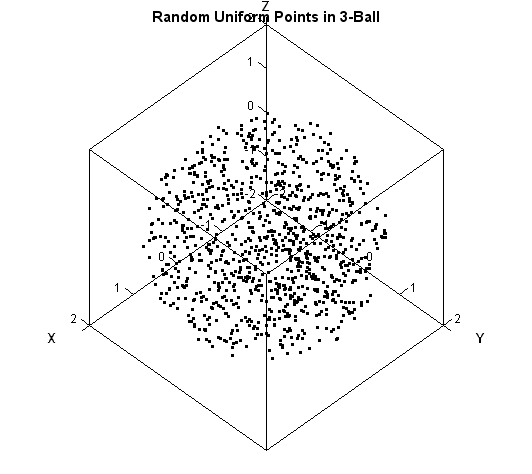Last week I showed how to generate random points uniformly inside a 2-d circular region. That article showed that the distance of a point to the circle's center cannot be distributed uniformly. Instead, you should use the square root of a uniform variate to generate 2-D distances to the origin. This result generalizes to higher dimensions. If you want to simulate points uniformly in the d-dimensional ball, generate the radius to be proportional to the dth-root of a uniform random variate.

Although it is possible to generalize the polar mapping and obtain a parameterization of the d-dimensional ball, there is an easier approach to simulate points in a ball. The basic idea is to simulate d independent standardized normal variables, project them radially onto the unit sphere, and then adjust their distance to the origin appropriately. You can find this algorithm in many textbooks (Devroye, 1986; Fishman, 1996), but Harman and Lacko (2010) summarize the process nicely. To paraphrase:

If Y is drawn from the uncorrelated multivariate normal distribution, then S = Y / ||Y|| has the uniform distribution on the unit d-sphere. Multiplying S by U1/d, where U has the uniform distribution on the unit interval (0,1), creates the uniform distribution in the unit d-dimensional ball.

It is easy to carry out this operation in SAS. In Base SAS, you can write a DATA step that uses arrays. The following program provides a SAS/IML solution. I used SAS/IML Studio to create a 3-D rotating scatter plot of the simulated cloud of points. You can download the SAS program that contains the DATA step and the IMLPlus program that creates the animation.

```proc iml; call randseed(12345); d = 3; /* dimension = number of variables */ N = 1000; /* sample size = number of obs */ radius = 2; /* radius of circle */ Y = randfun(N // d, "Normal"); /* Y ~ MVN(0, I(d)) */ u = randfun(N, "Uniform"); /* U ~ U(0,1) */ r = radius * u##(1/d); /* r proportional to d_th root of U */ X = r # Y / sqrt(Y[,##]); /* Y[,##] is sum of squares for each row */ /* X contains N random uniformly distributed points in the d-ball */```tags: IMLPlus, Simulation

The post Generate points uniformly inside a d-dimensional ball appeared first on The DO Loop.